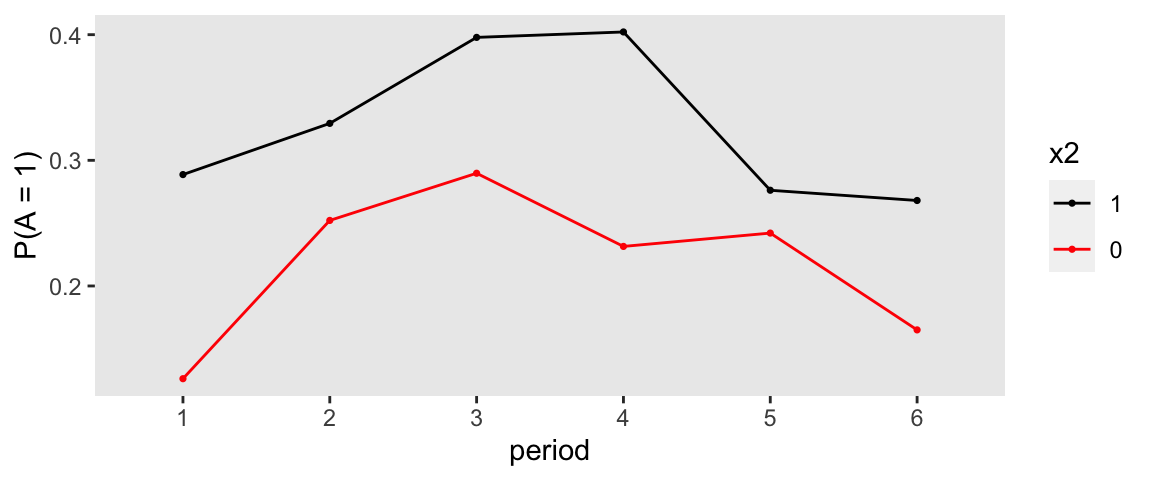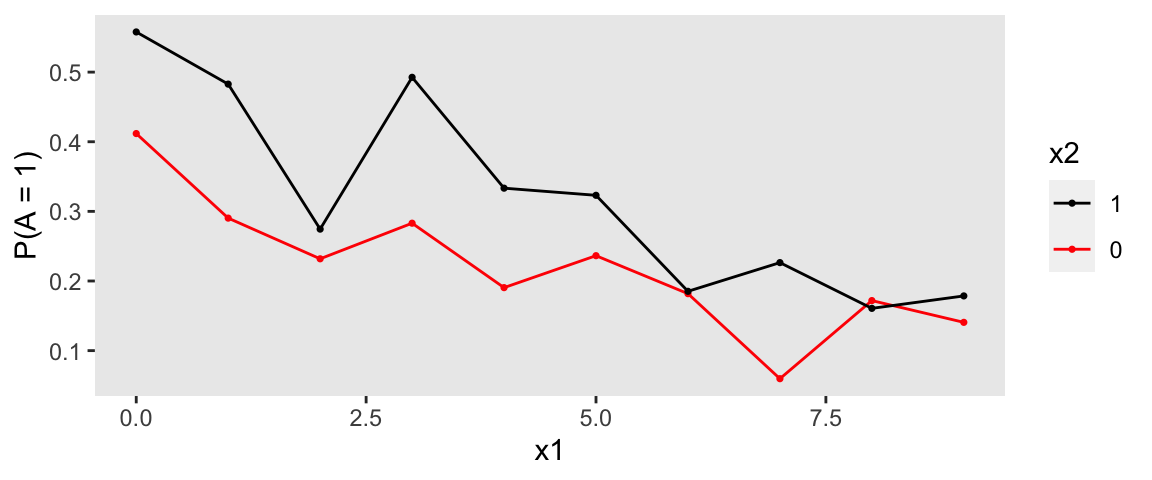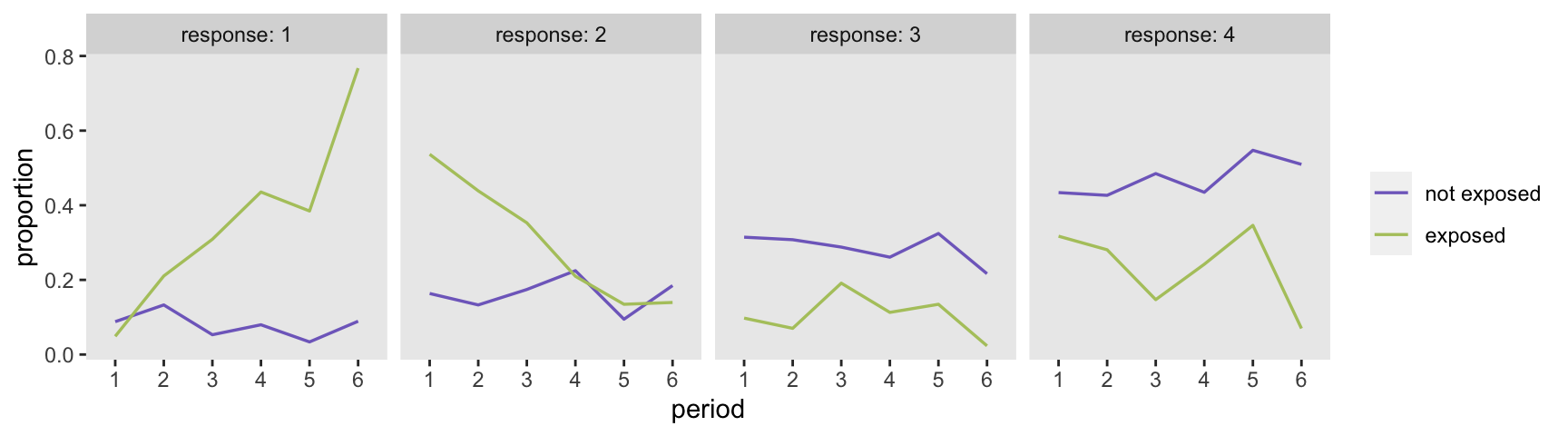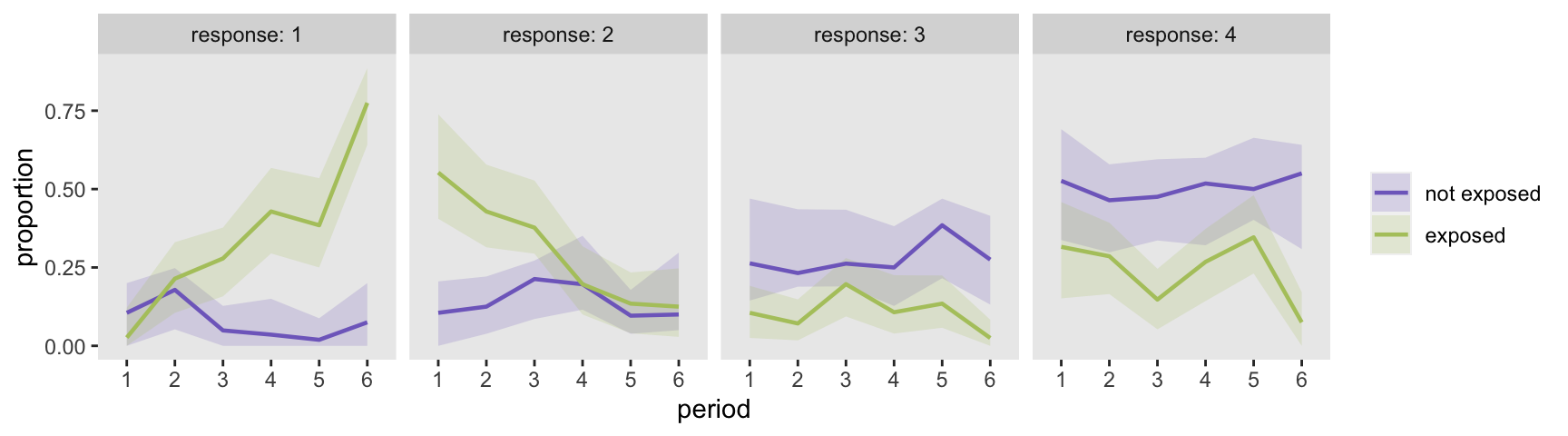# Presenting results for multinomial logistic regression: a marginal approach using propensity scores

Multinomial logistic regression modeling can provide an understanding of the factors influencing an unordered, categorical outcome. For example, if we are interested in identifying individual-level characteristics associated with political parties in the United States (Democratic, Republican, Libertarian, Green), a multinomial model would be a reasonable approach to for estimating the strength of the associations. In the case of a randomized trial or epidemiological study, we might be primarily interested in the effect of a specific intervention or exposure while controlling for other covariates. Unfortunately, interpreting results from a multinomial logistic model can be a bit of a challenge, particularly when there is a large number of possible responses and covariates.

My goal here is to generate a data set to illustrate how difficult it might be to interpret the parameter estimates from a multinomial model. And then I lay out a relatively simple solution that allows us to easily convert from the odds scale to the probability scale so we can more easily see the effect of the exposure on the outcome.

### The multinomial logistic model

This is the formal specification of the model:

$log\left[\frac{P(P_i = y)}{P(P_i = ref)}\right] = \alpha_y + \gamma_y A_i+ \boldsymbol{X_i \beta_y}$

where $$P_i$$ is the political party of individual $$i$$, $$P_i \in \{R, L, G\}$$ and ref is the reference party - we’ll set that to be $$D$$ in this case. $$A_i$$ is a treatment/exposure indicator, and is 1 if exposed, 0 otherwise. (Say there is a particular ad campaign that we are assessing.) $$\boldsymbol{X_i}$$ is a matrix of covariates.

The complexity of the model starts to emerge as we consider the parameters, which are all specific to the level of $$y$$. $$\alpha_y$$ is the intercept for the log odds of $$y$$ vs. the reference category (so $$\alpha_y$$ represents the log odds for the unexposed with average covariate values, assuming all covariates have been centered at zero). $$\gamma_y$$ is the log odds ratio comparing the odds of $$y$$ vs. $$D$$ for the exposed and unexposed. $$\boldsymbol{\beta_y}$$ is a vector of parameters that reflect the association of the covariates with the party choice.

If we have $$K$$ possible categories (in this example $$K = 4$$), there are $$K-1$$ sets of parameters. That is challenging enough. But each of those parameters is in relation to the reference category, so if you want to compare the odds across two non-reference categories, it can be a little challenging.

### Simulating the data

Before getting started, here are the necessary R packages for everything we are doing here.

library(simstudy)
library(data.table)
library(ggplot2)
library(nnet)
library(MatchIt)

#### Definitions

In this simulation, I am generating a categorical outcome that has four levels. There is a single exposure ($$A$$) and two covariates ($$x_1$$ and $$x_2$$). Six time periods worth of data are being generated, The probability of exposure ($$A$$) depends on both covariates and time. The outcome is associated with the covariates, and the effect of the intervention changes over time, complicating matters.

First, I define the effects of time on both exposure and the outcome (defT). Then I define covariates $$x_1$$ and $$x_2$$, exposure $$A$$, and outcome $$Y$$ (defY).

trunc_norm <- function(mean, sd, lower, upper) {
msm::rtnorm(n = 1, mean = mean, sd = sd, lower = lower, upper = upper)
}

defT <- defData(varname = "a_j",
formula = "trunc_norm(mean = 0, sd = 0.4,
lower = -Inf, upper = 0)", dist = "nonrandom")
defT <- defData(defT, varname = "b_j",
formula = "trunc_norm(mean = 0, sd = 0.4,
lower = -Inf, upper = 0)", dist = "nonrandom")

defY <- defDataAdd(varname = "x1", formula = 0, variance = 1)
defY <- defDataAdd(defY, varname = "x2", formula = 0.5, dist = "binary")
defY <- defDataAdd(defY, varname = "A", formula = "-1 + a_j - .5*x1 + .6*x2",
dist = "binary", link = "logit")
defY <- defDataAdd(defY, varname = "y",
formula = "b_j - 1.3 + 0.1*A - 0.3*x1 - 0.5*x2 + .55*A*period;
b_j - 0.6 + 1.4*A + 0.2*x1 - 0.5*x2;
-0.3 - 0.3*A - 0.3*x1 - 0.5*x2 ",
dist = "categorical", link = "logit")

#### Data generation

In the data generation step, we assume six periods and 200 individuals in each period:

set.seed(999)

dd <- genData(6, defT, id = "period")
dd <- genCluster(dd, "period", 200, level1ID = "id")

dd <- genFactor(dd, "y")

dd[, fy := relevel(fy, ref = "4")]
dd[, period := factor(period)]

Here are a few rows of the data set:

##       period   id A     x1 x2 fy
##    1:      1    1 0  0.101  0  3
##    2:      1    2 0  0.901  1  4
##    3:      1    3 0 -2.074  1  4
##    4:      1    4 1 -1.229  0  2
##    5:      1    5 0  0.643  1  4
##   ---
## 1196:      6 1196 0  0.164  1  1
## 1197:      6 1197 1 -1.571  1  1
## 1198:      6 1198 1  0.398  1  1
## 1199:      6 1199 0 -0.199  0  4
## 1200:      6 1200 0  1.022  1  1

Here are a two figures that allow us to visualize the relationship of the exposure $$A$$ with the covariates and time:Typically, I would like to plot the raw outcome data to get an initial sense of the how the outcome relates to the covariates of interest, but with a categorical measure that has four levels, it is not so obvious how to present the data in an insightful way. At the very least, we can show the distributions of the outcome over time and exposure, without taking into account the covariates (so there might be some confounding inherent in the plots):If we do suspect there might be confounding due to covariates $$x_1$$ and $$x_2$$, and we see that there appears to be different effects of exposure over time, it would not be unreasonable to estimate a multinomial model that adjusts for both covariates and includes an interaction term for exposure and period.

fit <- multinom(fy ~ x1 + x2 + A*period, data = dd, trace = FALSE)

#### Interpreting the results

The parameter estimates for a multinomial model are shown in the table below. In this case, the fourth response category is the reference, so the table shows the odds ratios for each response relative to the reference (the intercepts $$\alpha_y$$’s are not shown in the table). Each section of the table (labeled “1”, “2”, and “3”) represent the estimated parameters for each response level. While some readers may be able to get a lot out of this table, I find it a little overwhelming, particularly when it comes to understanding (a) the impact of time on the exposure effect, and (b) how responses other than the reference category compare to each other.

Characteristic 1 2 3
OR1 95% CI1 p-value OR1 95% CI1 p-value OR1 95% CI1 p-value
x1 0.70 0.57, 0.85 <0.001 1.16 0.97, 1.38 0.10 0.65 0.55, 0.76 <0.001
x2 0.68 0.46, 1.01 0.056 0.83 0.60, 1.15 0.3 0.65 0.48, 0.87 0.005
A 0.63 0.13, 3.16 0.6 5.28 2.28, 12.2 <0.001 0.34 0.10, 1.13 0.078
period
1
2 1.54 0.71, 3.34 0.3 0.81 0.41, 1.61 0.5 0.99 0.58, 1.71 >0.9
3 0.55 0.21, 1.46 0.2 0.94 0.49, 1.82 0.9 0.85 0.49, 1.47 0.6
4 0.88 0.37, 2.10 0.8 1.36 0.73, 2.55 0.3 0.81 0.46, 1.41 0.5
5 0.32 0.11, 0.94 0.038 0.46 0.22, 0.94 0.034 0.86 0.51, 1.46 0.6
6 0.91 0.40, 2.06 0.8 0.94 0.50, 1.75 0.8 0.63 0.36, 1.09 0.10
A * period
A * 2 3.32 0.52, 21.1 0.2 1.04 0.33, 3.33 >0.9 0.87 0.16, 4.60 0.9
A * 3 26.2 3.78, 182 <0.001 1.40 0.42, 4.67 0.6 5.34 1.18, 24.1 0.029
A * 4 14.0 2.22, 88.0 0.005 0.36 0.11, 1.17 0.089 2.00 0.42, 9.42 0.4
A * 5 27.4 3.87, 193 <0.001 0.44 0.12, 1.68 0.2 1.83 0.40, 8.44 0.4
A * 6 89.7 11.3, 714 <0.001 1.16 0.22, 6.14 0.9 2.02 0.15, 27.0 0.6
1 OR = Odds Ratio, CI = Confidence Interval

#### Probability scale

What I really want is to be able to see the estimates on the probability scale. This is always challenging because we can only get predicted probabilities at specific levels of the covariates (i.e. the model is conditional), and it is not clear what levels of the covariates we should use for this purpose. While we could just use the average value of each covariate to generate an average probability for each exposure group and each time period, there is something arbitrary to doing this.

Perhaps, a somewhat more palatable approach is get estimates of the marginal probabilities. A while ago, I presented an approach in the context of logistic regression (which is just a special case of multinomial regression, where the categorical outcome has only two levels) that estimated a predicted probability for each individual under each treatment arm, and then calculated an average risk difference by averaging across all the patients. This could presumably work here, but I decided to try another approach that eliminates the covariates from the analysis by using propensity score matching.

### Propensity score matching

I’ve described propensity score matching in an earlier post (and provided at least one good reference there), so I won’t go into much detail here. The general idea is that we can estimate a probability of exposure (i.e., the propensity score), and then match individuals in the two exposure groups based on those scores. If done well, this creates two comparable groups that are balanced with respect to the confounders (assuming all the confounders have been measured and are included in the exposure model for the propensity score). Once the matching is done, we can estimate the multinomial model without any covariates - and convert to predicted probabilities without relaying on any assumptions about the covariates.

In the first step, I am matching individuals within each time period This way, the groups will be balanced at each time point, and I could estimate marginal probabilities for each period.

matchby <- function(dx) {

m <- matchit(A ~ x1 + x2, data = dx,
method = "nearest", distance = "glm", caliper = .25)
match.data(m)

}

m.out <- rbindlist(lapply(1:6, function(x) matchby(dd[period==x])))

#### Analysis of matched data

In the second step, I fit a multinomial model that includes only exposure and time, and then generate predicted probability for each exposure and period combination.

mfit <- multinom(fy ~ A*period, data = m.out, trace = FALSE)

dnew <- data.table(expand.grid(A = c(0,1), period = factor(c(1:6))))
dpred <- data.table(predict(mfit, newdata = dnew, "probs"))

dpred <- cbind(dnew, dpred)
dplot <- melt(data = dpred, id.vars = c("A", "period"),
value.name = "proportion", variable.name = "response")
dplot[, response := factor(response, levels = c(1, 2, 3, 4))]
dplot[, A := factor(A, labels = c("not exposed", "exposed"))]

Here are the predicted probabilities for second time period:

##              A period response proportion
## 1: not exposed      2        1     0.1786
## 2:     exposed      2        1     0.2143
## 3: not exposed      2        2     0.1250
## 4:     exposed      2        2     0.4286
## 5: not exposed      2        3     0.2321
## 6:     exposed      2        3     0.0714
## 7: not exposed      2        4     0.4643
## 8:     exposed      2        4     0.2857

#### Bootstrap estimates of confidence bands

To go along with our point estimates, we need a measure of uncertainty, which we will estimate by bootstrap. For this analysis, I am bootstrapping the whole process, starting by sampling with replacement within each period and each exposure group, doing the matching, fitting the model, and generating the predictions.

bs <- function(x) {

ids <- dd[, .(id = sample(id, .N, replace = T)), keyby = .(period, A)][, id]
db <- dd[ids]
db[, id := .I]

mb.out <- rbindlist(lapply(1:6, function(x) matchby(db[period==x])))

mfit <- multinom(fy ~ A*period, data = mb.out, trace = FALSE)

dbnew <- data.table(expand.grid(A = c(0,1), period = factor(c(1:6))))
dbpred <- data.table(predict(mfit, newdata = dbnew, "probs"))

cbind(iter = x, dnew, dbpred)
}

bspred <- rbindlist(lapply(1:500, function(x) bs(x)))

#### Plot point estimates and confidence bands

What follows is the code to generate the figure showing the predicted probabilities for each arm. But before creating the plot, I’ve extracted 95% confidence intervals for each response level and period from the bootstrap data that will be used to draw the confidence bands.

bsplot <- melt(data = bspred, id.vars = c("iter", "A", "period"),
value.name = "proportion", variable.name = "response")
bsplot[, response := factor(response, levels = c(1, 2, 3, 4))]
bsplot[, A := factor(A, labels = c("not exposed", "exposed"))]

ci <- bsplot[,
.(l95 = quantile(proportion, 0.025), u95 = quantile(proportion, 0.975)),
keyby = .(response, A, period)
]

ggplot(data = dplot, aes(x = period,  group = A)) +
geom_ribbon(data = ci,
aes(ymin = l95, ymax = u95, fill = A),
alpha = .2)  +
geom_line(aes(y = proportion, color = A), size = .8) +
facet_grid(. ~ response, labeller = label_both) +
theme(panel.grid = element_blank(),
legend.title = element_blank()) +
scale_color_manual(values = c("#806cc6", "#b2c66c")) +
scale_fill_manual(values = c("#806cc6", "#b2c66c"))The point estimates mirror the marginal raw data plots quite closely, which is hardly surprising since we treated time as a categorical variable and the model is saturated. The benefit of doing the modeling is that we have generated estimates of uncertainty, and are in a position to make some inferences. For example, it looks like the exposure has an increasing effect on the probability of a level “1” response in the last three periods. Likewise, the effect of the exposure on the probability of a level “2” response was strongest in the first two periods, and then disappears. And there is too much uncertainty to say anything definitive about level “3” and “4” responses.